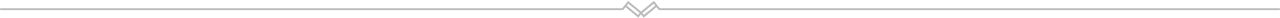# Watts and kWh 's the difference

The concepts of Watts and as in Power and KWh's explained.What do Watt and kWh mean?

The difference is quite simple, but it is easy to get confused.

Watts (W)
A watt (W) is a unit of measurement of power. Watts, therefore, refer to the power of your device.
Examples:
An incandescent lamp has a power of 60 W
A microwave oven has a maximum power of 900 W

The Wattage of the light bulb is the amount of energy it takes to produce a certain amount of light. The higher the wattage, the brighter the light, but also the more power it uses. ... 100 Watt incandescent lamp produces 1700 – 1800 lumens of “brightness” and uses 100 Watts of energy per hour.

Variations on watts:
1 kilowatt (kW) = 1,000 watts (W)
1 megawatt (MW) = 1,000 kilowatts (kW)

Handheld two-way radios use between 0.5-5 watts of power to broadcast a signal. Usually, a higher wattage allows for greater range and commands a higher price.
Handheld radios rely on batteries. How many watts you have affects the life of your batteries: the higher the wattage, the faster the batteries are drained

Determining how far you need your reception to reach will help you decide on how many watts you want for your handheld radio. The power of the radio is identified in watts. The wattage plays an important role in how far your transmission travels. Higher wattage generally means you have a farther reception range than lower wattage.

Watt is the unit of Power whereas kilowatt-hour (kWh) is the unit of Energy

Watt indicates the rate of using energy in Jules per second. You can compare this to how fast water is flowing out of a water pipe. If you have a Light bulb that has a rating of 100 watt, it means that the light bulb consumes 100 Jules per second.

kWh is the unit of energy. You can compare this to the volume of water that comes out of the pipe. A 100 watt light bulb, when used for an hour, will consume 100 Watt-hour of energy which is 0.1 kWh of energy.

Which will consume more electricity, a 60-watt bulb or a 100-watt bulb?

As stated, the standard unit of power is the watt, which is equal to one joule of energy per second. A secondary unit of energy is the kilowatt-hour (kWh), the equivalent of 1,000 watts of power for 1 hour.

So for example, if you are running both the bulbs for 2 hrs.
The energy consumed for 100-watt bulb will be 200 Wh or 0.2 kWh.

and for 60-watt bulb will be 120 Wh or 0.12 kWh.

So if for the same running time definitely, the more powerful bulb will consume more energy.

The Watt, as its many variations – kilowatt, megawatt, etc. – measures the electrical power of a device, whether it is a motor, a machine or the heating capacity of a boiler.
This information appears on the technical descriptions for all electrical appliances or should do.

The more powerful a device is, the higher the number of Watts.

If you multiply the total energy usage kWh with a charge per kWh (This should be on your electricity bill), you get your total Electricity cost.

Watt and Kilowatt-hour (kWh) practical example
Your electricity provider charges you 11 cents per kWh.
You have an Electric Heater that consumes 1500 watts (1.5kw). You use the Electric Heater for 3 hours every day.
For 3 hours it will cost you 1.5kw x 3 hours = 4.5kWh x .11 = .495 cents (50 cents)
Over a month it will cost you .495 x 30 days = 14.85

Written by:  - 5 Jan, 2019 (Viewed 2567 times)